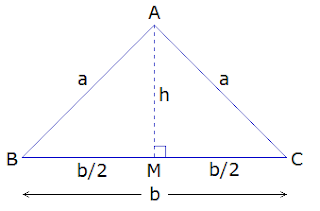# Isosceles Triangle

## Isosceles Triangle

A triangle with any two sides equal is called an isosceles triangle. The unequal side is known as the base, and the two angles at the ends of base are called base angles. And, the angle opposite to base is called the vertical angle.

In the given figure of triangle ABC, AB = AC, so it is an isosceles triangle. BC is the base, ABC and ACB are base angles and BAC is the vertical angle.

**********************

10 Math Problems officially announces the release of Quick Math Solver, an android APP on the Google Play Store for students around the world.**********************

### Area of an isosceles triangleLet ΔABC be an isosceles triangle. AB = AC = a, and the base BC = b. Then AMBC is drawn. Thus, AM = h and  BM = CM = b/2

### Properties (theorems) of isosceles triangle:

1.    Base angles of an isosceles triangle are equal.
2.    The bisector of vertical angle of an isosceles triangle is perpendicular to the base.
3.    The bisector of the vertical angle of an isosceles triangle bisects the base line segment.

### Theoretical proofs:

Theorem 1. “Base angles of an isosceles triangle are equal.”

Given: In triangle ABC, AB = AC
To prove: B = C
Construction: AMBC drawn.
Proof:
Statements                               Reasons
1.   In ΔABM and ΔACM
i.        AMB = AMC (R) -----> both being right angles (AMBC).
ii.      AB = AC (H) ---------------> given.
iii.    AM = AM (S) --------------> being common side.
2.    ΔABM ΔACM --------------> by R.H.S. axiom.
3.   ABM = ACM ------------> corresponding angles of triangles.
4.   B = C ------------------------> from statement 3.
Proved.

Note: The converse of theorem-1 is also true i.e. if two angles of a triangle are equal, then the sides opposite to them are equal.

Theorem 2. “The bisector of vertical angle of isosceles triangle is perpendicular to the base.”

Given: In ΔABC, AB = AC and AD is the bisector of vertical angle BAC i.e. BAD = CAD.
Proof:
Statements                            Reasons
1.    In ΔABD and ΔACD
i.        AB = AC (S) -------------> given.
2.    ΔABD ΔACD --------------> by S.A.S. axiom.
4.    ADBC ---------> being linear pair angles equal (statement 3.)
Proved.

Note: The converse of theorem-2 is also true i.e. if the line segment from vertex is perpendicular base then it bisects the vertical angle.

Theorem 3.The bisector of the vertical angle of an isosceles triangle bisects the base line segment.

Given: In ΔABC, AB = AC and AD is the bisector of vertical angle BAC i.e. BAD = CAD.
To prove: BD = DC
Proof:
Statements                             Reasons
1.    In ΔABD and ΔACD
i.        AB = AC (S) ----------------> given.
2.    ΔABD ΔACD -------------> by S.A.S. axiom.
3.    BD = DC -----------> corresponding sides of triangles.
Proved.

Note: The converse of theorem-3 is also true i.e. the line joining the vertex to mid-point of the base bisects the vertical angle.

### Workout Examples

Example 1: Find the angles indicated by x and y in the given figure.
Solution: From the figure,
x = 60° --------> base angles of isosceles Δ.

y + 60° = 90° --------> exterior angle of a Δ is equal to the sum of two opposite interior angles.
or,   y = 90° – 60°
or,   y = 30°

x = 60° and y = 30°

Example 2: Find the angles indicated by x and y in the given figure.
Solution: From the figure,
HTM = x ---------> being HT = HM
BHT = y -----------> being HT = BT
HTM = HBT + BHT ------> exterior angle of a Δ is equal to the sum of two opposite interior angles.
or,     x = y + y
or,     x = 2y ----------------(i)

x + y = 90° ------> exterior angle of a Δ is equal to the sum of two opposite interior angles.
or,     2y + y = 90° -----------> putting x = 2y from (i)
or,     3y = 90°
or,     y = 90°/3
or,     y = 30°

x = 2y
= 2 × 30°
= 60°

x = 60° and y = 30°

Example 3: Find the a, b, c, d and e from the given figure.
Solution: From the figure,
a = 37° --------> base angles of isosceles Δ.

a + b + 37° = 180° --------> sum of angles of a Δ.
or,    37° + b + 37° = 180°
or,    b + 74° = 180°
or,    b = 180° – 74°
or,    b = 106°

c + b = 180° ---------> linear pair of angles.
or,    c + 106° = 180°
or,    c = 180° – 106°
or,    c = 74°

d = c -----------> base angles of isosceles Δ.
= 74°

74° + 74° + e = 180° ------> sum of angles of a Δ.
or,    148° + e = 180°
or,    e = 180° – 148°
or,    e = 32°

a = 37°, b = 106°, c = 74°, d = 74° and e = 32°

You can comment your questions or problems regarding isosceles triangle here.